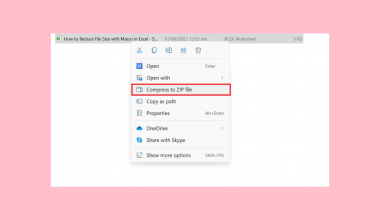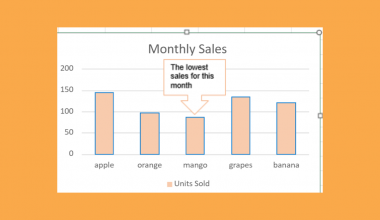# How to Keep Leading Zeros in Excel CSV

This guide will explain how to keep leading zeros in Excel CSV using two simple methods.

CSV or a Comma Separated Values is a plain text file containing a list of data in a specific format. CSV saves the data in a table structured format. We can also use it with any spreadsheet program.

Excel is an extremely powerful and valuable tool containing numerous built-in features and functions that make our work easier. But there are times when Excel does not work the way we want it to.

Excel changes the format of our data, especially when transferred and opened in another program. For instance, Excel removes the leading zeros in Excel CSV. This often happens when the CSV is opened in an Excel file.

Since Excel interprets the leading zeros as unnecessary and changes the format, it will remove the leading zeros. And this will return a completely different data.

Let’s take a scenario where this situation may happen.

Suppose you received a CSV file from a colleague. And you opened the file using Microsoft Excel. Let’s say the file contained postal codes which had leading zeros. But the leading zeros were removed when opened in Excel.

This is because Excel changes the format to general, which removes the leading zeros. If you checked the original file and the opened file in Excel, you would see that the leading zeros disappear in the postal code. So this gives you different data.

To keep the leading zeros in Excel CSV, there are two ways we can do this. One way is to change the number format of the data to the correct one and keep the leading zeros.

Another way is to use the `TEXT` function. The `TEXT` function will allow us to keep the leading zeros by converting our data to a specific text format. In this case, we will follow the format “00000” to keep leading zeros.

Before we begin learning how to keep leading zeros in Excel CSV, let’s dissect the `TEXT` function.

## The Anatomy of the TEXT Function

The syntax or the way we write the `TEXT` function is as follows:

`=TEXT(value;format_text)`

Let’s take this apart and understand what each term means:

• = how we start any function or equation in Excel.
• TEXT() is our `TEXT` function. The `TEXT` function converts any numeric data to a text given a specific number format.
• value is referring to the number we want to change the format.
• format_text refers to our given number format.

Awesome! Now let’s learn a real example of keeping the leading zeros in Excel CSV.

## A Real Example of Keeping Leading Zeros in Excel CSV

For instance, we receive a CSV file containing information on the different branch office. One information is the zip code of each branch office. When we open the CSV file in Excel, the leading zeros in the zip code are removed.So we want to keep the leading zeros for accuracy purposes. To do this, we will first utilize the first method, which is to change the number format. Since this information is specifically talking about zip code, we can simply choose the zip code format available in the Number Format category.

If it was a different data and we want to keep the leading zeros, we can choose the Custom category. From the Custom category, we will choose the ‘00000’ format to keep the leading zeros.

Furthermore, we can also use the second method, which utilizes the `TEXT` function. So we simply use the `TEXT` function to format the data to ‘00000’ and apply it to the data.

And these two methods will allow us to keep the leading zeros in our Excel CSV.

Awesome! Now let’s learn the steps of how to keep leading zeros in Excel CSV.

## How to Keep Leading Zeros in Excel CSV

In this section, we will learn the process of how to keep leading zeros in Excel CSV using the two methods.

1. Firstly, let’s try the first method. Simply select or highlight the column containing the data you want to keep the leading zeros of. Then, right-click and select Number Format.2. Secondly, we will change the format. Since the data is a zip code, we can simply go to Category and select Special. Under the Type, we will select Zip Code. Finally, click OK to apply the changes.Furthermore, if you are not based in the United States, you can change your location to get the appropriate Zip code format in the Locale (location) dropdown menu.

3. Alternately, we can go to the Custom category. Under the Type menu, scroll to the very bottom and select 00000. Then, click OK to apply the changes.4. And tada! We have kept the leading zeros in our Excel CSV file.5. Furthermore, let’s try another method using the `TEXT` function. First, create another column to input the fixed data. Then, type an ‘=’ sign and ‘TEXT’ to start the function. Next, select the cell containing the data you want to keep the leading zero of, which in this case is D3. Lastly, input the format ‘00000’.

So the entire formula will be ‘=TEXT( ,”00000”)’. Finally, press the Enter key to return the result.6. Next, drag down the formula to copy it to the rest of the column.7. And tada! We have changed the data format and kept the leading zeros in our Excel CSV.That’s pretty much it! So you have learned how to keep leading zeros in Excel CSV using two methods. Hence, now you can make use of any of the two methods to retain the zeros in a CSV file and keep the accuracy of data.

Are you interested in learning more about what Excel can do? You can now use the various other Microsoft Excel formulas available to create great worksheets that work for you. Make sure to subscribe to our newsletter to be the first to know about the latest guides and tutorials from us.Our goal this year is to create lots of rich, bite-sized tutorials for Google Sheets users like you. If you liked this one, you'll love what we are working on! Readers receive ✨ early access ✨ to new content.

##### You May Also Like## How to Calculate Standard Error in Excel

This guide will discuss how to calculate the standard error in Excel using two easy methods. Excel is…## How to Reduce File Size with Macro in Excel

This guide will discuss three easy and simple methods on how to reduce file size with macro in…## How to Calculate NormalCDF Probabilities in Excel

We can use the NORM.DIST function to calculate NormalCDF probabilities in Excel. The NORM.DIST function allows users to…## How to Do 3D Interpolation in Excel

This guide will explain how to do 3D interpolation in Excel. Linear interpolation refers to a method used…